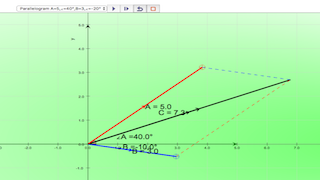## Topics

Measurement of length and time

## Description

A vector is a quantity that has both magnitude and direction. Displacement, velocity, acceleration, and force are the vector quantities. in simpler examples, vectors were simply directed up, down, left or right. In situations in which vectors are directed at angles to the customary x(horizontal)-y(vertical) coordinate axes, a useful mathematical trick will be employed to transform the vector into two parts with each part being directed along the coordinate axes. What if the customary x-y coordinate axes is not (horizontal) and (vertical)? Sometimes, it could be useful to resolve vectors along and perpendicular to a slope, direction of travel than always horizontal and vertical,

## What is shown here?

1. What are the x and y components of the RED vector A?
2. record down on a piece of paper.
3. What are the x and y components of the BLUE vector B?.
4. record down on a piece of paper.
5. now, you have the values of (Ax, Ay) = _______ and (Bx, By) = _______

## How to calculate the resultant of 2 vectors?

1. click PLAY to visualise the meaning of addition of vector A with B.
2. What are the components of this vector sum?
3. How do they relate to the components of the original (RED and BLUE) vectors?
4. The vector (BLACK) sum C = (Cx, Cy) is now a vector that reaches from the tail of the first (RED) A = (Ax,Ay) vector to the head of the second (BLUE) B = (Bx,By) vector.
5. test yourself whether you have enough practice calculating C = (Cx,Cy).

## Other interesting fun activities

1. this model can be used to calculate any A & B vectors.

## Sample Learning Goals

(e) state what is meant by scalar and vector (how to resolve) quantities and give common examples of each
(f) calculate vector by means of components in horizontal and vertical axes.

## Version:

### Translations

Code Language Translator Run### Software Requirements

SoftwareRequirements

 Android iOS Windows MacOS with best with Chrome Chrome Chrome Chrome support full-screen? Yes. Chrome/Opera No. Firefox/ Samsung Internet Not yet Yes Yes cannot work on some mobile browser that don't understand JavaScript such as..... cannot work on Internet Explorer 9 and below

### CreditsThis email address is being protected from spambots. You need JavaScript enabled to view it.

### end faq

http://iwant2study.org/lookangejss/01_measurement/ejss_model_horizontalverticalsum/horizontalverticalsum_Simulation.xhtml

### Learning Design

London Knowledge Lab - Institute of Education

http://learningdesigner.org/viewer.php?uri=/personal/lookang/public/submissions/fid/ea027fd9dc3544126bd69930fe3d29ffe41e8fc9329134346474481680b6178b

### Topics

Measurement of length and time

### Description

A vector is a quantity that has both magnitude and direction. Displacement, velocity, acceleration, and force are the vector quantities. in simpler examples, vectors were simply directed up, down, left or right. In situations in which vectors are directed at angles to the customary x(horizontal)-y(vertical) coordinate axes, a useful mathematical trick will be employed to transform the vector into two parts with each part being directed along the coordinate axes. What if the customary x-y coordinate axes is not (horizontal) and (vertical)? Sometimes, it could be useful to resolve vectors along and perpendicular to a slope, direction of travel than always horizontal and vertical,

### What is shown here?

1. What are the x and y components of the RED vector A?
2. record down on a piece of paper.
3. What are the x and y components of the BLUE vector B?.
4. record down on a piece of paper.
5. now, you have the values of (Ax, Ay) = _______ and (Bx, By) = _______

### How to calculate the resultant of 2 vectors?

1. click PLAY to visualise the meaning of addition of vector A with B.
2. What are the components of this vector sum?
3. How do they relate to the components of the original (RED and BLUE) vectors?
4. The vector (BLACK) sum C = (Cx, Cy) is now a vector that reaches from the tail of the first (RED) A = (Ax,Ay) vector to the head of the second (BLUE) B = (Bx,By) vector.
5. test yourself whether you have enough practice calculating C = (Cx,Cy).

### Other interesting fun activities

1. this model can be used to calculate any A & B vectors.

### Sample Learning Goals

(e) state what is meant by scalar and vector (how to resolve) quantities and give common examples of each

(f) calculate vector by means of components in horizontal and vertical axes.

## Version:

### Practice

http://goo.gl/forms/2ITP4YvbZp

[text]

[text]

### Version:

1. http://weelookang.blogspot.sg/2016/02/vector-addition-b-c-model-with.html improved version with joseph chua's inputs
2. http://weelookang.blogspot.sg/2014/10/vector-addition-model.html original simulation by lookang

### Other Resources

1. http://ophysics.com/k11.html by This email address is being protected from spambots. You need JavaScript enabled to view it.

### end faq

4.8888888888889 1 1 1 1 1 1 1 1 1 1 Rating 4.89 (9 Votes)
Parent Category: Physics
Category: 01 Measurements
Hits: 11708